# Intersection of sets - math problems

#### Number of problems found: 63

• Subtracting sets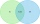For two sets K, L is true: K has 30 elements, L has 27 elements and the set L - K has 22 elements. How many elements does the set K - L have?
• DisjointHow many elements have union and intersection of two disjoint sets when the first have 1 and secodn 8 elements.
• The triangleThe triangle is given by three vertices: A [0.0] B [-4.2] C [-6.0] Calculate V (intersection of heights), T (center of gravity), O - center of a circle circumscribed
• Two sets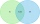Suppose Set B contains 69 elements and the total number elements in either Set A or Set B is 124. If the Sets A and B have 29 elements in common, how many elements are contained in set A?
• Operations with sets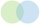The set B - A has twice as fewer elements than the set A - B and four times fewer elements than the set A ∩ B. How many times more elements does the set A have than the set B?
• Coordinates of the intersection of the diagonals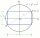In the rectangular coordinate system, a rectangle ABCD is drawn. The vertices of the rectangle are determined by these coordinates A = (2.2) B = (8.2) C = (8.6) D = (2.6) Find the coordinates of the intersection of the diagonals of the ABCD rectangle
• Probability of intersectionThree students have a probability of 0.7,0.5 and 0.4 to graduated from university respectively. What is the probability that at least one of them will be graduated?
• Trousers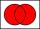In the class was 12 students. Nine students wearing trousers and turtleneck eight. How many students worn trousers with a turtleneck?
• Glasses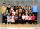Imagine a set of students in your class (number of students: 19), who wears glasses. How much minimum and maximum element may contain this set.
• Line intersect segment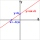Decide whether the line p : x + 2 y - 7 = 0 intersects the line segment given by points A[1, 1] and B[5, 3]
• Dices throwsWhat is the probability that the two throws of the dice: a) Six falls even once b) Six will fall at least once
• Sphere in coneA sphere is inscribed in the cone (the intersection of their boundaries consists of a circle and one point). The ratio of the surface of the ball and the contents of the base is 4: 3. A plane passing through the axis of a cone cuts the cone in an isoscele
• Wafers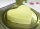They used 200 pieces to make wafers. 157 topped with caramel topping and 100 with sugar topping. How many wafers did both toppings have?
• Round destiny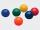There are 5 white and 10 red balls in the destiny. 4 balls will be drawn at random. What is the probability of the event "at least 2 spheres are white"?
• Open intervals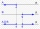Open intervals A = (x-2; 2x-1) and B = (3x-4; 4) are given. Find the largest real number for which A ⊂ B applies.
• Perpendicular projectionDetermine the distance of a point B[1, -3] from the perpendicular projection of a point A[3, -2] on a straight line 2 x + y + 1 = 0.
• Small painters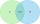There are ten pupils in the painting group. Eight pupils paint with watercolors and nine pupils with tempera colors. How many pupils paint both water and tempera colors when each pupil paints?
• PupilsThere are 27 pupils in the classroom. They can swim 21 and ski nine pupils. Neither swim nor ski three pupils. How many pupils can swim and ski?
• Fall sum or sameFind the probability that if you roll two dice, it will fall the sum of 10, or the same number will fall on both dice.
• The box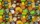The box contains five chocolate, three fruit, and two menthol candies. We choose sweets at random from the box. What is the probability that we will take out one chocolate, one fruit, and one menthol candy without a return?

Do you have an interesting mathematical word problem that you can't solve it? Submit a math problem, and we can try to solve it.

We will send a solution to your e-mail address. Solved examples are also published here. Please enter the e-mail correctly and check whether you don't have a full mailbox.

Please do not submit problems from current active competitions such as Mathematical Olympiad, correspondence seminars etc...